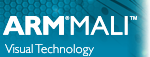OpenGL ES SDK for Android ARM Developer Center
Tutorials

Note
Finished versions of all of the tutorials listed are included in the SDK. See Building Android samples for instructions on how to use them.

## Tutorials

These tutorials introduce the basics of an OpenGL ES application. The source for these samples can be found in the folder of the SDK.

Summary of changes between versions of the OpenGL ES SDK for Android.

1. First Android Native Application

An introduction and walkthrough of Android native applications.

A quick introduction to the programmable graphics pipeline introduced in OpenGL ES 2.0.

3. Graphics Setup

4. Simple Triangle

How to create your first triangle.

5. Simple Cube

Introduction in transformations and movement in OpenGL ES 2.0.

6. Texture Cube

How to start texturing your objects to make them look realistic.

7. Lighting

Basic lighting using OpenGL ES 2.0.

8. Normal Mapping

Basic Normal Mapping tutorial that uses Normal Maps.

Using the Open Asset Importer to load models into OpenGL ES.

10. Vertex Buffer Objects

How to use Vertex Buffer Objects to reduce the bandwidth in your application.

How to package assets up into the apk and load files from the file system.

12. Mipmapping and Compressed Textures

This tutorial introduces the idea of mipmapping and compressed textures.

13. Projected Lights

Projected Lights effect using OpenGL ES 3.0.

14. Bloom

Bloom effect using OpenGL ES 3.0.

15. Min Max Blending

The application demonstrates behaviour of blending in GL_MIN and GL_MAX mode in OpenGL ES 3.0.

16. Integer Logic

The application simulates cellular automata phenomenon following Rule 30 using OpenGL ES 3.0.

17. ETC2 Texture

Demonstration of ETC2 texture compression support in OpenGL ES 3.0.

18. Boids

Demonstration of Transform Feedback functionality in OpenGL ES 3.0.

Demonstration of shadow mapping functionality using OpenGL ES 3.0.

20. Occlusion Query

Demonstration of Occlusion Query functionality in OpenGL ES 3.0.

21. Instanced Tessellation

The application displays a rotating solid torus with a low-polygon wireframed mesh surrounding it. The torus is drawn by means of instanced tessellation technique using OpenGL ES 3.0.

22. Instancing

This sample presents the instanced drawing technique using OpenGL ES 3.0.

23. Using multiview rendering

This sample presents the GL_OVR_multiview and GL_OVR_multiview2 extensions and how they can be used to improve performance for virtual reality use cases.

These samples show optimised implementations of more complex algorithms. The source for these samples can be found in the folder of the SDK.

• Texture Compression and Alpha Channels

This document describes the related samples "ETCAtlasAlpha", "ETCCompressedAlpha", and "ETCUncompressedAlpha", which illustrate three different ways of handling alpha channels when using ETC1 compression.

• High Quality Text Rendering

Improving quality for textured text.

Illustrates the use of sync objects to synchronise the use of shared objects between multiple contexts in multiple threads.

• Metaballs

Using a GPU to create organic-looking 3-dimensional objects in OpenGL ES 3.0.

• Terrain Rendering with Geometry Clipmaps

This sample will show you how to efficiently implement geometry clipmaps using OpenGL ES 3.0. The sample makes use of 2D texture arrays as well as instancing to efficiently render an infinitely large terrain. The terrain is asynchronously uploaded to the GPU using pixel buffer objects.

• Skybox

This sample presents how to implement skybox using single cubemap texture.

This sample uses OpenGL ES 3.0 and Pixel Local Storage to perform advanced shading techniques. The sample computes a per-pixel object thickness, and uses it to render a subsurface scattering effect for translucent geometry, without the use of external depth-maps or additional rendertargets.

• ASTC textures

This document describes usage of compressed ASTC textures.

• ASTC low precision

This document describes how to enable and use the ASTC decode mode extension to select decoding precision when decoding ASTC image blocks.

This document will give you an introduction to compute shaders in OpenGL ES 3.1, how they fit into the rest of OpenGL ES and how you can make use of it in your application. Using compute shaders effectively requires a new mindset where parallel computation is exposed more explicitly to developers. With this explicitness, various new primitives are introduced which allows compute shader threads to share access to memory and synchronize execution.

• Particle Flow Simulation with Compute Shaders

This sample illustrates how to efficiently perform calculations on a large amount of particles using OpenGL ES 3.1 and compute shaders.

• Occlusion Culling with Hierarchical-Z

This sample will show you how to efficiently implement occlusion culling using compute shaders in OpenGL ES 3.1. The sample tests visibility for a large number of instances in parallel and only draws the instances which are assumed to be visible. Using this technique can in certain scenes give a tremendous performance increase.

• Ocean Rendering with Fast Fourier Transform

This sample will show you how to efficiently implement high quality ocean water rendering using compute shaders in OpenGL ES 3.1.

• Displacement mapping with tessellation

This sample uses OpenGL ES 3.1 and the Android extension pack to perform displacement mapping with tessellation. The sample investigates common techniques used to improve performance and visuals.

• Procedural modelling with geometry shaders

This sample uses OpenGL ES 3.1 and the Android extension pack to procedurally generate complex geometry in real-time with geometry shaders.

The rest of the advanced samples are summarised in Advanced OpenGL ES Samples